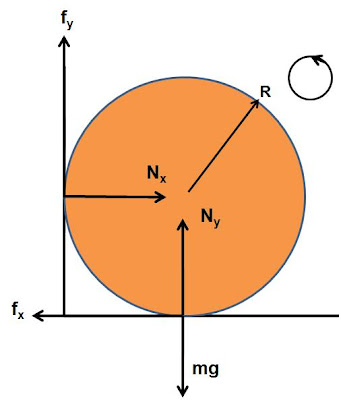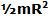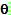## Friday, November 14, 2008

### Irodov Problem 1.248There are two surfaces of contact for the cylinder, i) the vertical wall and ii) the floor. Both these surfaces offer a force of friction to resist the slipping of the cylinder relative to them. Since the cylinder is spinning in the anti-clockwise direction when it was initially placed, the force of friction on each of these surfaces will oppose its relative motion and so the friction force from vertical wall fy will act upwards while the friction force fx from the surface will act to the left as shown in the figure. Let Nx and Ny be the normal reactions acting from the vertical and horizontal wall respectively. Let the mass of the cylinder be m.

There are three forces acting on the body in the vertical direction, i) the force of gravity mg acting downwards, ii) the normal reaction from the floor Ny acting upwards and iii) the force of friction Ny from the vertical wall. Since there is no accelaration for the cylinder in the upward direction we have,In the horizontal direction there are two forces acting on the cylinder, i) the force of friction fx from the floor and ii) the normal reaction from the vertical wall Nx. Since the cylinder is not accelerating in the horizontal direction we have,Now from our basic understanding of friction force is Nk and so we have,From (1), (2), (3) and (4) we obtain,The two friction forces from the wall and the floor result in a resistive torque slows the rotating cylinder and this torque is simply equal to (fx + fy)R. Since the moment of inertia of the cylinder about its axis of rotation isits angular deceleration is given by,If at some instant of time the angular velocity of the cylinder is, and it has turned an anglethen we have,Since in the end the cylinder stops completely its final angular velocity is 0, so from (10) we have,Since each complete rotation means that the cylinder has turned byradians. If the cylinder turns an angleradians then the number of rotations its undergoes is. Hence the total number of rotations that the cylinder will undergo before it stops will be,